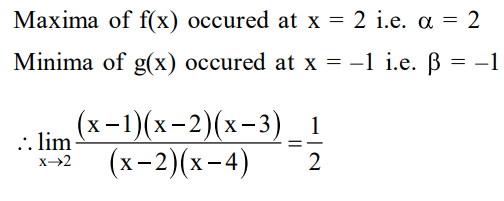# Prove the following identities.`
Question:

Let $f(x)=5-|x-2|$ and $g(x)=|x+1|, x \in R$.

If $f(x)$ attains maximum value at $\alpha$ and $g(x)$

attains minimum value at $\beta$, then

$\lim _{x \rightarrow-\alpha \beta} \frac{(x-1)\left(x^{2}-5 x+6\right)}{x^{2}-6 x+8}$ is equal to :

1. $1 / 2$

2. $-3 / 2$

3. $3 / 2$

4. $-1 / 2$

Correct Option: 1

Solution: